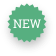# Big Game: Goldman Sachs' Elephant Hunt in Libya

Published 24 Sep 2018
Reference 6274
Topic
Industry
Region
Length 10 page(s)
Summary

The case focuses on the ill-fated relationship between the LIA, Libya’s new sovereign wealth fund, and Goldman Sachs, and the ultimately disastrous \$1.2 billion derivatives (elephant) trades the LIA entered into in early 2008 on Goldman’s advice. The analysis deals with basic derivative instruments, terminology and concepts (e.g., leverage, counterparty risk) as well as valuation issues both intuitive (e.g., put-call parity, arbitrage-based valuation bounds) and technical (binomial trees, Black-Scholes formula, Monte Carlo simulations, volatility and dividend yield calibration). It also discusses the pricing and hedging of exotic derivatives. Epilogue: In a subsequent lawsuit brought by the LIA, Goldman Sachs was accused of having exploited the lack of finance acumen of LIA staff to lure them into trades whose riskiness they did not understand. In October 2016, a London court ruled against the LIA.

Teaching objectives

The main issues covered: • Basic derivative instruments: forwards, puts, calls, and combinations thereof. • Payoff diagrams, with and without the effect of prices and trade fees. • Key concepts in derivatives (e.g., leverage effect, counterparty risk). • The impact of maturity, strike price and early exercise on a derivative’s value. • The put-call parity relationship. • Arbitrage-based valuation bounds. • The valuation of derivatives using binomial trees, the Black-Scholes formula and Monte Carlo simulations. • Volatility and dividend yield calibration. • Pricing and hedging exotic derivatives Target Audience: • Requiring only a basic knowledge of derivatives, the case is suited to undergraduates, MBAs or executives, as a core course introduction to derivatives or the first session of an elective. Students with a basic knowledge of the binomial model can also learn how to adjust it to handle dividends and exotic options, and how to calibrate parameters using input options. • The case covers both the basics of options (definitions, payoffs, etc.) and valuation (binomial tree, Black-Scholes). It can be taught as part of a longer course in different configurations: • One session: “option basics” at the start of a course as an introduction to options • Two sessions: “option basics” at the start of a course, “valuation” towards the end • One session: “valuation” going quickly over option basics • Two sessions: both “option basics” and “valuation” in practice as a wrap-up on options • It can also be the sole case in a short course on options. Session 1 requires a lecture on option basics and Session 2 a lecture on binomial tree valuation (and possibly one on Black-Scholes).

Keywords
• derivatives: forwards, puts, calls
• exotic options
• Put-Call Parity
• option valuation
• binomial model
• Black-Scholes formula
• dividends
• volatility
• leverage
• risk
• counterparty risk
• lawsuit
• sovereign wealth fund
• derivatives mis-selling
• Payoff diagrams
• Impact of maturity, strike price and early exercise on a derivative’s value
• Arbitrage-based valuation bounds
• binomial trees
• Volatility estimation
• Dividend yield calibration
• Monte Carlo simulations
• Hedging
• Speculation
• Toxic instruments
• Q11819